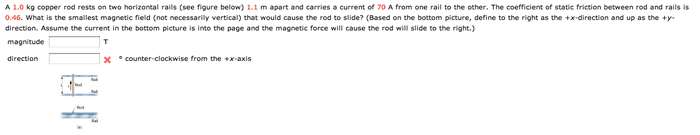# Magnetic fields

## Homework Statement## Homework Equations

this is what i think i figured out to be the equation for force as a function of θ:

F(θ) = (μmg)/(cos(θ) + μsin(θ))

## The Attempt at a Solution

I tried to find extrema using the numbers given to figure out the angle, but the number i am getting is not right. This is kind of a last ditch effort as I need to finish this within a half hour